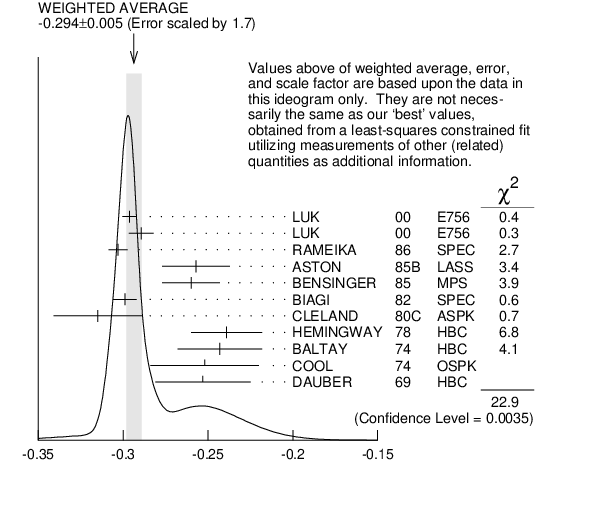${{\boldsymbol \Xi}^{-}}$ DECAY PARAMETERS

See the Note on Baryon Decay Parameters'' in the neutron Listings.

$\alpha\mathrm {({{\boldsymbol \Xi}^{-}})}{}\alpha _{−}({{\boldsymbol \Lambda}}$)

VALUE EVTS DOCUMENT ID TECN  COMMENT
 $\bf{ -0.294 \pm0.005}$ OUR AVERAGE  Error includes scale factor of 1.7. See the ideogram below.
$-0.2963$ $\pm0.0042$ 189k
 2000
E756 ${{\mathit p}}$ Be, 800 GeV
$-0.2894$ $\pm0.0073$ 63k 1
 2000
E756 ${{\mathit p}}$ Be, 800 GeV
$-0.303$ $\pm0.004$ $\pm0.004$ 192k
 1986
SPEC 400 GeV ${{\mathit p}}{}^{}\mathrm {Be}$
$-0.257$ $\pm0.020$ 11k
 1985 B
LASS 11 ${\mathrm {GeV/}}\mathit c$ ${{\mathit K}^{-}}{{\mathit p}}$
$-0.260$ $\pm0.017$ 21k
 1985
MPS 5 ${\mathrm {GeV/}}\mathit c$ ${{\mathit K}^{-}}{{\mathit p}}$
$-0.299$ $\pm0.007$ 150k
 1982
SPEC SPS hyperon beam
$-0.315$ $\pm0.026$ 9046
 1980 C
ASPK BNL hyperon beam
$-0.239$ $\pm0.021$ 6599
 1978
HBC 4.2 ${\mathrm {GeV/}}\mathit c$ ${{\mathit K}^{-}}{{\mathit p}}$
$-0.243$ $\pm0.025$ 4303
 1974
HBC 1.75 ${\mathrm {GeV/}}\mathit c$ ${{\mathit K}^{-}}{{\mathit p}}$
$-0.252$ $\pm0.032$ 2436
 1974
OSPK 1.8 ${\mathrm {GeV/}}\mathit c$ ${{\mathit K}^{-}}{{\mathit p}}$
$-0.253$ $\pm0.028$ 2781
 1969
HBC
 1 This LUK 2000 value is for $\alpha\mathrm {({{\overline{\mathit \Xi}}^{+}})}$ $\alpha _{+}({{\overline{\mathit \Lambda}}}$). We assume $\mathit CP$ conservation here by including it in the average for $\alpha\mathrm {({{\mathit \Xi}^{-}})}$ $\alpha _{−}({{\mathit \Lambda}}$). But see the second data block below for the $\mathit CP$ test.$\alpha\mathrm {({{\mathit \Xi}^{-}})}{}\alpha _{−}({{\mathit \Lambda}}$)
References:
 LUK 2000
PRL 85 4860 Search for Direct $\mathit CP$ Violation in Nonleptonic Decays of Charged ${{\mathit \Xi}}$ and ${{\mathit \Lambda}}$ Hyperons
 RAMEIKA 1986
PR D33 3172 Measurement of Production Polarization and Decay Asymmetry for ${{\mathit \Xi}^{-}}$ Hyperons
 ASTON 1985B
PR D32 2270 Inclusive Production of Multistrange Hyperons from 11 ${\mathrm {GeV/}}\mathit c$ ${{\mathit K}^{-}}{{\mathit p}}$ Interactions
 BENSINGER 1985
NP B252 561 Measurement of Decay Parameters and Polarization in Inclusive ${{\mathit \Xi}^{-}}$ Production from ${{\mathit K}^{-}}{{\mathit p}}$ Interactions
 BIAGI 1982
PL 112B 265 Determination of the $\alpha$ Parameter for the Decay ${{\mathit \Xi}^{-}}$ $\rightarrow$ ${{\mathit \Lambda}}{{\mathit \pi}^{-}}$
 CLELAND 1980C
PR D21 12 Studies in the BNL 21 ${\mathrm {GeV/}}\mathit c$ Negative Hyperon Beam. I. ${{\mathit \Sigma}}$ and ${{\mathit \Xi}}$ Production and Asymmetry in Decay ${{\mathit \Xi}^{-}}$ $\rightarrow$ ${{\mathit \Lambda}}{{\mathit \pi}^{-}}$
 HEMINGWAY 1978
NP B142 205 ${{\mathit \Omega}^{-}}$ Produced in ${{\mathit K}^{-}}{{\mathit p}}$ Reactions at 4.2 ${\mathrm {GeV/}}\mathit c$
 BALTAY 1974
PR D9 49 Properties of ${{\mathit \Xi}^{-}}$ and ${{\mathit \Xi}^{0}}$ Hyperons
 COOL 1974
PR D10 792 Measurement of the Magnetic Moment and of the Decay Parameters of the ${{\mathit \Xi}^{-}}$ Hyperon
 DAUBER 1969
PR 179 1262 Production and Decay of Cascade Hyperons